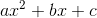Search

# Algebraic Expansion and Factorization (GCSE/14+)

Updated: Apr 3, 2020Expanding single brackets

To expand a bracket means to multiply each term of the brackets to the term outside of the brackets. For example in the expression 2(a+4), multiply both a and 4 by 2, so

2(a+4) = 2 x a + 2 x 4 = 2a+8.

Expanding double brackets

To expand double brackets, we simply perform the above procedure but we treat the bracket outside as a single term first. For example in the expression (2+b)(a+4), multiply both (a+4) by 2 and b, then we expand each single bracket.

So,

(2+b)(a+4) = 2 x (a+4) + b x (a+4) = 2 x a + 2 x 4 + b x a + b x 4 = 2a + 8 + ba + 4b

= 2a+4b+ab+8

We collect any like terms (if any) after expanding the brackets. Usually, the answers are usually written with descending order of powers.

Factorization

Factorization is the reverse of expanding brackets. The first step of factorization is to take out any common factors which have the terms have. For example in the expression ab+b, since b goes into both terms, you would write b(a+1).

There is a simple method that could work to factorise most quadratic expressions (if they can be factorised).We find two numbers that multiply to give ac and add to give b. It helps to list the factors of ac, and then trying to adding some to get b.

For example in the expressionSo we have to find two numbers that multiply to give 2 x 3 = 6 and the two numbers need to add to give 7.

The factor of 6 is 1,2,3,6. We know that 1 + 6 = 7. So we have found the two numbers.

Rewriting 7x with 6x + x, we could factorise the remaining expression easily.Factorization is an important way of solving quadratic equations. There are many ways to factorise a quadratic expression but they require sufficient practice before they become easier.

Anglo Academy has prepared you a free maths worksheet for you to practise your algebraic skills.

If you have any question or comment, please feel free to leave your comment below.